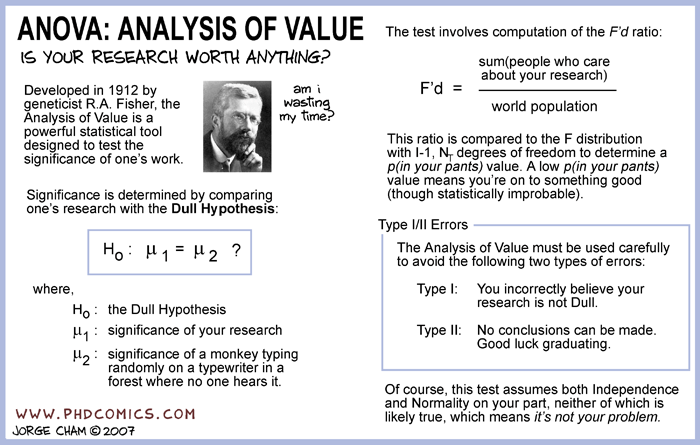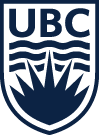### When should you ask Questions?

• Ya know the drill.... Sit With Your Proper Groups
•### Exams and stuff

I know that you have been studying. Try to relax between this lab and your exam. I totally believe in all y'all. (Assuming that you have been studying.) You got this!

### What is statistical independence

Two events are independent if the occurrence of one does not affect the probability of occurrence of the other. Similarly, two random variables are independent if the realization of one does not affect the probability distribution of the other.a

Q2a $$P(HAD)=.25$$ $$P(NHD)=.75$$ $$P(HD|E)=.25$$ $$P(NHD|E)=.75$$ $$P(HD|NE)=.25$$ $$P(NHD|NE)=.75$$